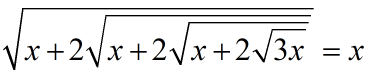Tuesday, November 24, 2020
Home > Latest Announcement > Number System Questions With Solutions Q No 41 To Q No 60

# Number System Questions With Solutions Q No 41 To Q No 60# Number System Questions With Solutions Q No 41 to Q No 60

In this post, you will get some important Number System Questions with Solutions Q No 41 To Q No 60. Questions will be solved soon.

If you can solve these Number Systems Question with Solutions Q No 41 To Q No 60. then please send us the solutions on my contact email: amansmathsblogs@gmail.com.

Ques No 41:

What is the unit digit of N, if N = (289)5 x (587)7 x (1156)9 x (17)15

Options:

A. 8

B. 5

C. 3

D. 6

Solution:

Ques No 42:

The numbers 1 to 29 are written side by side as follows 1234567891011……2829. If the number is divided by 9, then what is the remainder?

Options:

A. 0

B. 1

C. 2

D. 3

Solution:

Ques No 43:

The smallest integral value of x, for whichis an integer is

Options:

A. 1

B. -1

C. 7

D. -7

Solution:

Ques No 44:

The sum of the digits of two digit number is 11, if the digits are reversed the number decreases by 45 The number is

Options:

A. 38

B. 68

C. 74

D. 83

Solution:

Ques No 45:

If a2 – b2 = 13 where a and b natural numbers, then value of a is

Options:

A. 6

B. 7

C. 8

D. 9

Solution:

Ques No 46:

If 562 – 492 = 7p, then p is equal is

Options:

A. 115

B. 95

C. 105

D. 104

Solution:

Ques No 47:

When 75% of a two-digit number is added to it, the digits of the number are reversed. Find the ratio of the unit’s digit to the ten’s digit in the original number

Options:

A. 1:2

B. 1:4

C. 2:1

D. 3:2

Solution:

Ques No 48:

How many zeroes will be there in the expansion of the expression 11×22×33×44……..×100100?

Options:

A. 1200

B. 1232

C. 1300

D. 1296

Solution:

Ques No 49:

The number obtained by interchanging the two digits of a two digit number is less than the original number by 27. If the difference between the two digits of the number is 3, what is the original number?

Options:

A. 74

B. 63

C. 85

D. Can’t be determined

Solution:

Ques No 50:

If x is a positive integer such that 2x + 12 is perfectly divisible by ‘x’, then the number of possible values of ‘x’ is

Options:

A. 2

B. 5

C. 6

D. 12

Solution:

Ques No 51:

If A =, B =, C = (0.3)2, D = (-1.2)2, then

Options:

A. A > B > C > D

B. D > A > B > C

C. D > B > C > A

D. D > C > A > B

Solution:

Ques No 52:

The unit digit in the expression (36234)(33512)(39180)(5429)–(25123)(31512) will be

Options:

A. 8

B. 0

C. 6

D. 5

Solution:

Ques No 53:

Find the value of x inOptions:

A. 1

B. 3

C. 6

D. 12

Solution:

Ques No 54:

xy is a number that is divided by ab where xy < ab and gives a result 0. Xyxyxy. . . then ab result

Options:

A. 11

B. 33

C. 99

D. 66

Solution:

Ques No 55:

V is product of first 41 natural numbers. A = V + 1. The number of primes among A + 1, A + 2, A + 3, A + 4………………A + 39, A + 40 is

Options:

A. 1

B. 2

C. 3

D. 0

Solution:

Ques No 56:

If n is integer such that n x n = n + n, then the number of such number n, is

Options:

A. 0

B. 1

C. 2

D. 3

Solution:

Ques No 57:

Given that a, b are odd and c, d are even, then

Options:

A. a2 – b2 + c2 – d2 is always divisible by 4

B. abc + bcd + cda + dab is always divisible by 4

C. a4 + b4 + c3 + d3 + c2b + a2b is always odd

D. a + 2b + 3c + 4d is odd

Solution:

Ques No 58:

A positive number whose reciprocal equals one less than the number, is

Options:

A.B.C.D.Solution:

Ques No 59:

Which is one of the numbers listed below is not a divisor of the number N = (230 – 1), is equal to

Options:

A. 25 – 1

B. 25 + 1

C. 26 – 1

D. 210 + 1

Solution:

Ques No 60:

The remainder when (x51 + 51) is divided by (x + 1), is:

Options:

A. 0

B. 1

C. 51

D. 50

Solution:

error: Content is protected !!